# Need for Non-Dimensional Numbers

The importance of experiments in Fluid Mechanics needs no additional emphasis. Experiments are required in design and testing of vehicles such as aeroplanes, ships and automobiles, pumps, turbines, fans and other equipment. We also have experiments which are carried out from the point of view of understanding a flow and fundamental phenomena such as turbulence. Needless to say that the experiments have to be planned and executed methodically. The question we must ask ourselves is :"What are the parameters involved in the given flow? What is their relative significance?" These are some of the questions facing one that conducts any experiment. At the same time, presentation of results in a concise fashion also requires thought and planning. A thoughtless experiment (or for that matter a modern computation) will generate a large body of numbers. It may be difficult to interpret them. A thoughtful presentation will help to render them in a concise and understandable manner. It is here that Dimensional Analysis helps us.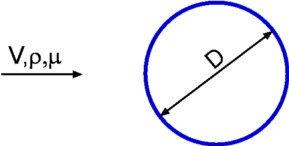Figure 5.1: Flow past a Cylinder

Let us consider a concrete situation of one trying to study drag for the flow about a cylinder. The first question is "What are the parameters that govern this process". The answer is well known - Freestream Speed V, Diameter of the cylinder D, density of the fluid,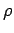and viscosity of the fluid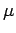. If one proceeds without thought one will want to determine the influence of each of the parameters. First one will perform a set of experiments varying free stream speed and keep the other parameters constant. One curve is produced for Drag vs V, keeping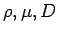, the same. But note that the same experiment has to be repeated for different values of these parameters as well. (Fig.5.2). Then one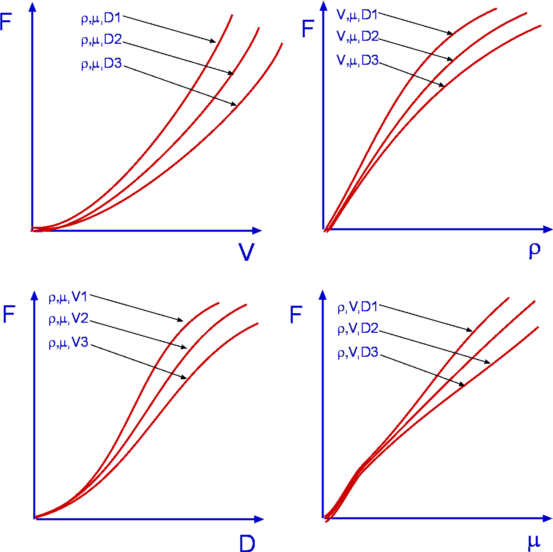Figure 5.2: Dependence of Drag on Various Parameters

has to consider drag vskeeping other parameters constant. In the end the whole exercise will become unruly. If each curve requires about ten points for a good definition, it is easy to see that as many as 10,000 experiments are needed, perhaps requiring one's lifetime?

Luckily, there is a method by which the number of such experiments could be reduced to a minimum. This is by determining the Non-Dimensional Numbers for the flow. It is somewhat ironical that we carry out a dimensional analysis to determine the non-dimensional numbers for the flow. These non-dimensional numbers involve certain combinations of the parameters involved (in the case of drag about cylinder these are drag force, F,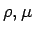,D,V). During the experiments, the strategy is to vary only these non-dimensional numbers. For example, we will see that for the drag problem the non-dimensional numbers are the drag coefficient Cd and Reynolds number Re (see Fig.5.3), which are given by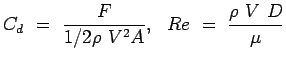(5.1)

While carrying out the experiment one need to vary only the Reynolds number. How this Reynolds number is varied is left to the investigator and his or her limitations. It may not be found possible to change the density or viscosity of the working fluid. Instead it may be easier to conduct the experiment for different speeds , but still the Reynolds number varies. Alternately it may be the diameter of the cylinder that can be easily varied.Figure 5.3: Variation of Cd with Re.

The second advantage of expressing the results in terms of these non-dimensional numbers becomes evident now. For this experiment the results are given as drag coefficient, Cd as a function of the Reynolds number Re. The user calculates the Reynolds number for his conditions and finds out what the value of Cd is and then works out the actual value of drag. A certain compactness is what is achieved.

Another advantage of non-dimensional numbers is that the results are independent of the units of measurement. So a person who is used to a totally different system of units can still interpret the results.

A further application of these numbers is in Similitude. Invariably whenever new vehicle or any such application is built it is to be tested out in the laboratory. Many times, it so happens that one cannot test the prototype in the laboratory. This could be because of the size as in the case of aeroplane or automobile, or due to the difficulty in obtaining the flow conditions as in the case of spacecraft. The usual practice is to fabricate a model of the prototype and test it in the laboratory. The question asked is "What should be the relationship between the model and the prototype? Is it enough to have a geometrical similarity between the two? Are there any more requirements?". Non-dimensional numbers answer this question. It will be seen that a few of the non-dimensional numbers will have to be equal between the model and the prototype.

For any process the Buckingham Pi Theorem helps determine the non-dimensional numbers. This theorem is stated in the next section.

(c) Aerospace, Mechanical & Mechatronic Engg. 2005
University of Sydney